# Multi-Octave 4-Channel Tone Synthesis With Magnitude Select

#### Loren Robinson - Loren@jhu.edu    Max Sa'pereira - msapere1@jhu.eduMatt McKee - Mckee@jhu.edu    Agatha Monzon - cyber_aggie@hotmail.comGraduate Advisor: Yunbin Deng - Yunbin@jhu.edu## Objectives

Tone creation and synthesis is an important part of Digital Signal Processing and has many applications. In our implementation, up to four notes from a single octave can be 'played' at a time, each in its respective channel. In addition, each channel has support for three different magnitudes. The digital part of the design creates the desired tone frequency in a square wave, which is sent to the analog part to be added to the other channels. This result is then sent through a low-pass Butterworth filter to remove as much of the harmonics as possible and create a sum of sine waves output.

## A Little Theory

Using Fourier Series expansion, it can be shown that a square wave is actually the sum of a signal and its odd harmonics. Each of the harmonics has a decreasing magnitude, inversely proportional to which harmonic it is. The following picture shows this, using only the first four odd harmonics in demonstration. The addition of more odd harmonics brings the composite waveform nearer the shape of a perfect square wave. A perfect square wave is, therefore, composed of an infinite number of odd harmonics.Odd sine harmonics sum to create a square wave

Using a low pass filter it is possible to remove all of the extra harmonics and get the original frequency sine signal out. This is made easier by the fact that each successive harmonic is a fraction of the original and only the odd harmonics exist. By using a Butterworth filter we are able to achieve a slight increase in magnitude of the original wave and an even further reduction of the odd harmonics.

For a more in-depth discussion, see our References section .

## Specifications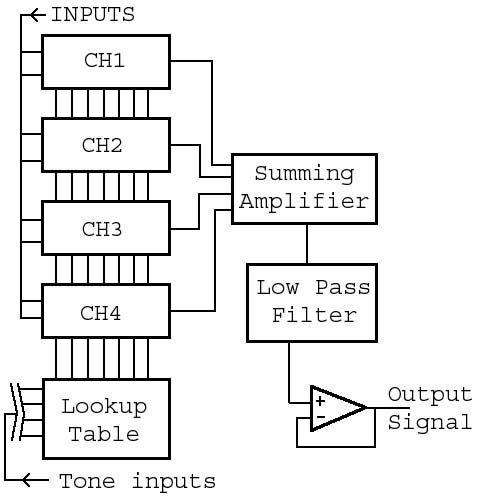Top level schematic of chip

Ultimately, our chip can be broken down into two parts: a digital and an analog part. The digital part consists of input logic, registers, counters, comparators, the lookup table and the 'glue' to interface with the analog part. The analog part is the summing amplifier for the four input channels, the Butterworth low pass filter and the final op-amp voltage follower acting as a buffer for the output signal.

Chip Pinout
 1 Vref - on Butterworth filter (2.5V) 40 Vbias - on Summing Amplifier (1.0V) 2 Vn - Bias Volt. on NMOS trans. in summing Amp. (3.0V) 39 Vout - Output signal 3 Vp - Bias Volt. on PMOS trans. in summing Amp. (2.0V) 38 VbiasOUT - on op-amp voltage follower (1.0V) 4 Vda - Analog voltage source (5.0V) 37 N.C. 5 Gnd 36 N.C. 6 Q3 - for Butterworth filter, wc = 1000Hz (453.1mV) 35 N.C. 7 Q2 - for Butterworth filter, wc = 500Hz (422.5mV) 34 N.C. 8 Q1 - for Butterworth filter, wc = 250Hz (392.4mV) 33 N.C. 9 g3 - for Butterworth filter, wc = 1000Hz (476.3mV) 32 N.C. 10 g2 - for Butterworth filter, wc = 500Hz (446.7mV) 31 N.C. 11 g1 - for Butterworth filter, wc = 250Hz (415.8mV) 30 N.C. 12 V1 - small magnitude voltage (0.5V) 29 N.C. 13 V2 - normal magnitude voltage (1.0V) 28 T3 - part of tone select 14 V3 - large magnitude voltage (2.0V) 27 T2 - part of tone select 15 Vdd - Digital voltage source (5.0V) 26 T1 - part of tone select 16 OCT1 - part of octave select 25 T0 - part of tone select 17 OCT0 - part of octave select 24 N.C. 18 CH0 - part of channel select 23 WRITE - write input data to selected channel 19 CH1 - part of channel select 22 CLK - 120KHz clock 20 AMP1 - part of tone magnitude select 21 AMP0 - part of tone magnitude select
Numbers in parenthesis are nominal voltages. They may be modified post fabrication to achieve the desired operation

#### Digital:

There are 12 pins that act as the digital input to this chip. Ten of them are used to describe what type of tone is to be generated, one is used for the input clock and the last pin is used for a write signal. Their operations are described in the following tables:

 AMP1 AMP0 Description CH1 CH0 Description 0 0 Turn off channel 0 0 Write to Channel 1 0 1 Magnitude = V1 0 1 Write to Channel 2 1 0 Magnitude = V2 1 0 Write to Channel 3 1 1 Magnitude = V3 1 1 Write to Channel 4

 OCT1 OCT0 Description 0 0 One octave above middle 0 1 Middle Octave 1 0 One octave below middle 1 1 Not defined

 T3 T2 T1 T0 Note 0 0 0 0 B 0 0 0 1 A#/Bb 0 0 1 0 A 0 0 1 1 G#/Ab 0 1 0 0 G 0 1 0 1 F#/Gb 0 1 1 0 F 0 1 1 1 E 1 0 0 0 D#/Eb 1 0 0 1 D 1 0 1 0 C#/Db 1 0 1 1 C

We chose [131 - 247]Hz to be one octave below middle, [262 - 494]Hz to be the middle octave, and [523 - 988]Hz to be one octave above middle. The middle A was chosen to be 440Hz according to current standards. Each of our octaves went from C to B. In the music world this means our three octaves are C1 to B2, C2 to B3, and C3 to B4. The actual frequency values we used can be found in a chart here: .

In order to achieve a square wave of proper frequency with a 50% duty cycle, we modified an idea found in an IEEE paper: . The basic idea is to divide the clock frequency by the desired frequency and use this number as a value that a counter will count up to. These values are stored in a lookup table according to the table listed above. The actual count values were determined for the highest frequency octave because it would have the most potential error. The lower and middle octaves use the same count values, but have external clock splitters and internal logic to decide which clock it uses. A comparator is used to compare the counter value with the desired value in the lookup table. When the two values are equal, the comparator will output a clock pulse and reset the counter. These pulses go into a flip flop set up as a clock splitter which will create a clock output with a 50% duty cycle every two pulses.

To choose an appropriate clock frequency, we went onto Digikey's website and found the cheapest and most common frequencies on the crystals it sold. We found about eight different common frequency values from 40KHz to 250Khz, and wrote a simple matlab program to determine which frequency would have the least squared error in approximating the highest octave's frequencies. The frequency with the least error turned out to be 120Khz, so we designed our lookup table with that value in mind.

Each 'channel' has a 7-bit counter, 8-bit comparator and 8-bit register. The counter only needs 7 bits because in order to have the proper frequency output, the lowest order bit is the clock itself, effectively making it an 8-bit counter. The 8-bit register just stores the value of the count value found in the lookup table every time the channel is written to. Each channel also has another four flip flops to hold data on the octave and amplitude of each note, which are used to determine which clock input to take and the magnitude of the tone for the summing amplifier.Layout of each channel - with counter, comparator and registers

#### Analog:

The analog portion of this project can be further split into two parts as well: the summing amplifer and the Butterworth low pass filter. All of the important biasing and reference voltages for the analog parts are off chip. This is so that if any unforseen problems occur, such as much higher than expected intrinsic capacitances being formed for example, these values will need to be changed to produce the desired operations.` `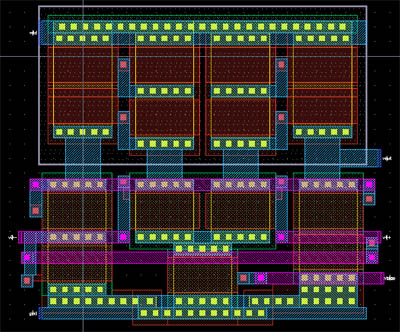Layout of Op-Amp Schematic of Op-Amp

Our implementation of the Op-amp used in the summing amplifier and the low pass filter was a modified version of one given in the notes. We just cascoded the current mirrors and made the transistors wider to support higher current flow.` `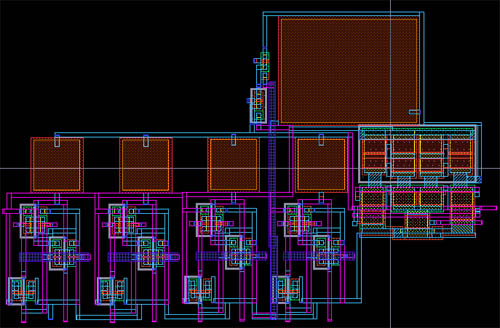Schematic of Summing Amplifier Layout of Summing Amplifier

The summing amplifer we used was also similar to one given in the class notes. Each wave input will have its own frequency and amplitude, so we need to add the individual amplitudes at their corresponding frequency rates. There are two pass gates per tone, operated at the output clock frequency of each channel, which decide the input to the summing amplifier. If the clock is high on a particular channel, the appropriate voltage (given by the tone's magnitude, stored in each channel) is applied to the input. If the clock is low, then Gnd is provided to the input.

Following the passgates is a set of four capacitor "banks," one for each channel, which store the charge accumulated over a clock pulse. On the output, charge redistribution occurs, so that all the voltage developed over each branch will balance across all four branchs and yield one fourth of the total charge sum. This voltage then connects to the negative terminal of the next part of summing amplifier, the transconductance amplifier (a "sense amplifier").

Input into the transconductance amplifier is the average charge summation at the negative terminal, and the reference voltage Vref at the positive terminal. Vref is the voltage over which the output waveform will be centered. For viewing purposes we chose Vref = 2.5V so the output wave would be centered over a range of about 0 to 5.0V.

The feedback of the trans. amp. is a capacitor in parallel with a resistor. Resistors are notoriously difficult to implement in CMOS and maintain a linear transfer characteristic along with not taking up too much space. The solution was to use an NMOS and PMOS transistor in series. The gates of these are attached to pins and have bias voltages of Vref plus/minus 0.5V respectively. The resistor must be there to allow an actual DC voltage change at Vout. The capacitor only passes the AC portion of the wave, so without both of these components the summation could not occur.

In general this circuit can be seen as an inverting amplifier with gain Cf (for the feedback capacitor) divided by 4Ci (equivalent capacitance of four capacitors in parallel at the input). The output in our case is the sum of the input tone amplitudes times the gain added to a reference voltage. The output of this is then applied to the low pass filter: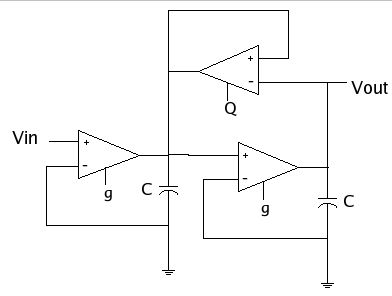Schematic of 2nd order low pass filter ` `Layout of 2nd order low pass filterDerivation of Transfer Function ` ` To make this a Butterworth low pass filter, the first thing we did was write down the transfer function of this schematic. This is derived using the simple rules associated with operational amplifiers discussed in our notes and in class.The definition of a butterworth filter describes the transfer function of the filter as having two poles with negative real parts. They are complex and are located at -135o and +135o in the complex plane. So to avoid oscillation and have a real butterworth filter we had to fit our equation to this constraint.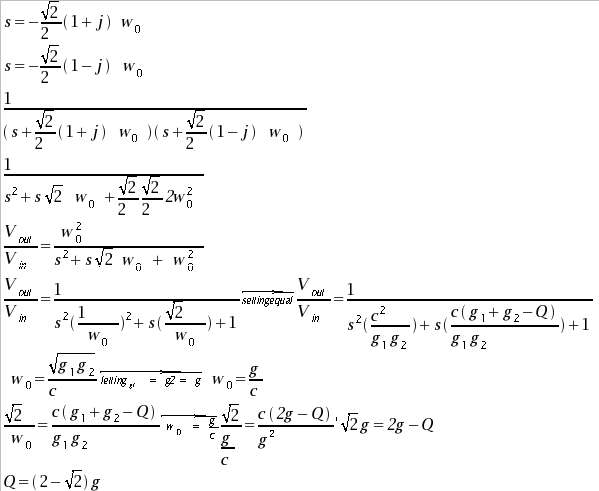Derivation of g to Q relationship ` ` Using the -135o and +135o pole location definition of a Butterworth filter, we solved a general second order transfer function in terms of s and its conjugate. We then set this value equal to the result obtained from the derivation of the transfer function of our schematic and determined how the g and Q were related. We determined that Q is related to g by a factor of two minus the square root of two.
The next step was determining the transconductance values (g and Q) for each cutoff frequency (fc). These values were obtained by using the equations
(1) w=g/c
(2) w=2*Pi*fc
(3) g = dI/(v2-v1)
in conjunction with the transconductance amplifier characteristic plots of Iout vs (v2-v1), and Iout as function of Vbias.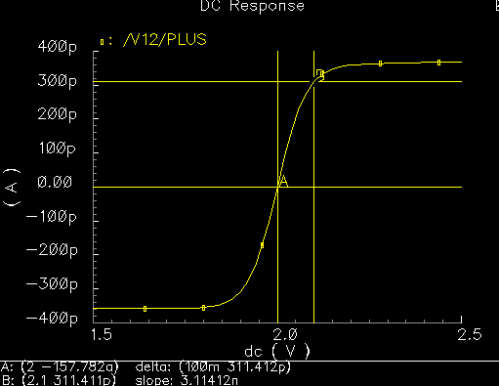` ` Here we plotted Iout as a function of v2. We went about this plot by fixing v1 at 2V and then sweeping v2. From this graph we observe the slope. The slope corresponds to our transconductance value. What we aim for here is a large range over which the slope (transconductance value) is constant. Our inputs will depend on our tone amplitudes, values over which we have little control. Therefore to be safe we played with values until we reached a comfortable 0.1V deviation range. Basically this means that as long as the difference between our inputs (to trans amp) lie within the 0.1V range, the lowpass filter will work correctly. Solving for dI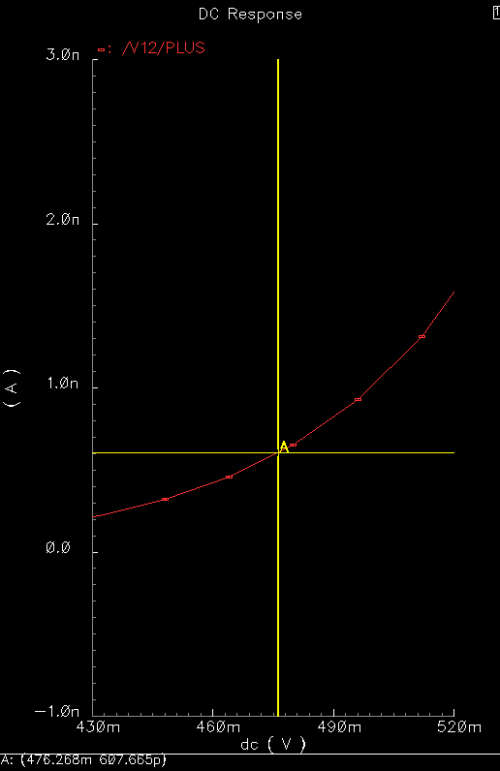` ` Using this dV and the relationship g=dI/dV, we then solve for I. Now all we do is find the Vbias corresponding to I. This is achieved by observing the Iout vs. Vbias relationship of our transconductance. We trace our predetermined I to the plotted curve. Our corresponding Vbias is the one we want. Solving for Vbias
The Vbias solved for in the last step will be the g values we want for each of the respective cutoff frequencies in the low pass filter. We then solve for Q using the relationship found earlier. All these values are located in the pinout table.

## Results

The digital and analog parts of the chip were tested seperately to assure proper operational behavior, then attached together on the chip. To prevent unwanted operation of the analog components, we chose to use two voltage sources. A digital source which would tolerate a certain amount of bounce and wiggle, and a 'clean' analog source with a nice steady value. The grounds were chosen to be the same, but only after surrounding all the digital parts with a bulk and metal connection to ground. This should prevent the ground bounce characteristic of rapidly switching digital components from excessively affecting the analog parts of our chip.` `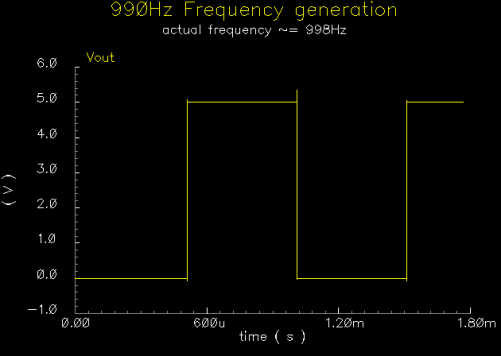932Hz generated tone 990Hz generated tone

The previous graphs show two of the possible frequencies generated by the digital part of the chip. These are two of the highest frequencies the chip will generate, and as such have the highest possible error and take the least amount of time to simulate. As it was, each of these two plots took about 25 minutes to simulate. We assume that since these two frequencies output correctly and the logic controlling the clock input works correctly, the other frequencies will generate correctly as well.500Hz Transfer Characteristic

This is the transfer characteristic of the butterworth low pass filter for 500Hz. It has a peak at 500Hz and a fairly sharp decline up to about 2000Hz.` `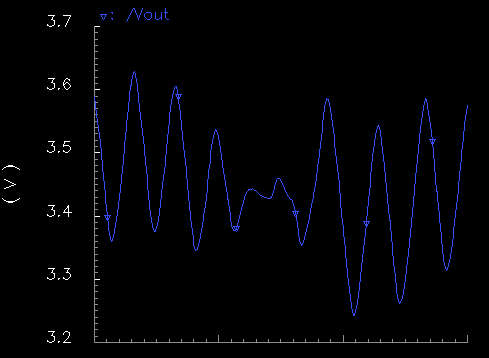Matlab Result Circuit Result

Adding up two frequencies, 880Hz and 990Hz, we get the above waveform. On the left is the 'ideal' waveform graphed with Matlab, and on the right is the waveform that our chip produces. As can easily be seen the results are very similar.` `Matlab Result Circuit Result

This is the sum of three frequencies, 880Hz, 932Hz and 990Hz. Also, the 932Hz wave is half the magnitude of the other two, as given with our implementation; i.e., the 932Hz wave uses V1 as its magnitude voltage and the other two use V2 as their magnitude voltage. This output doesn't quite follow the one from Matlab as closely as the previous one, especially near the middle. Over a larger period of time, the waveform does look more similar to the 'ideal' waveform. There is also a noticeable phase shift on the signals from the chip which causes some of the discrepancy. However, phase shifting a tone doesn't affect the overall sound to a human ear so this output is acceptable.

## References

 Abdullah, T. Yahay, "Music Scales - Frequency, Notes, Octaves, ...," http://tyala.freeyellow.com/4scales.htm

 Wittman, B. A.; Early, S.H.; Messerschmitt, D.G., "A Hardware Multitone Digital Frequency Synthesizer", IEEE Transactions on Acoustics, Speech, and Signal Processing, Vol. ASSP-27, No. 6, December 1979. pgs 804-809.

 Higgins, Anna-Marie, "Fourier Synthesis" http://www.clubi.ie/amhiggins/fourier1.html